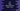# C# program to find the sum of all numbers from 1 to n## C# program to find the sum of all numbers from 1 to n:

In this C# program, we will learn how to find the sum of all numbers from 1 to n. It will take the value of n as input from the user and print out the sum to the user.

This program can be solved by using a loop. In this post, we will learn how to find the sum by using a for loop and by using a while loop. Each loop will run from 1 to n and print out the sum at the end.

### By using a for loop:

Let’s first use a for loop to solve this. Below is the complete algorithm this program will use:

• Initialize one variable as 0 to store the total sum.
• Read the value n as user-input from the user.
• Run one for loop from 1 to n.
• Inside the loop, adds the current value of the for loop to the sum variable. At the end of the program, print the value of sum.

C# program that uses the above algorithm:

``````using System;

public class Program {

public static void Main(string[] args) {
int i, n, sum = 0;
Console.WriteLine("Enter the value of n :");

n = Convert.ToInt32(Console.ReadLine());

for(i = 1; i<= n; i++){
sum += i;
}

Console.WriteLine("Total sum: "+sum);
}
}``````

Here,

• sum is the variable to hold the total sum. It is initialized as 0.
• The value of n is taken as user-input.
• The for loop runs from i = 1 to i = n. Inside the loop, it is adding the value of i to sum.
• Finally, it is printing the value of sum.

It will print the below output:

``````Enter the limit :
5
Total sum: 15``````

### By using a while loop:

We can also use a while loop to find the sum. Below is the complete program:

``````using System;

public class Program {

public static void Main(string[] args) {
int i = 1, n, sum = 0;
Console.WriteLine("Enter the value of n :");

n = Convert.ToInt32(Console.ReadLine());

while(i <= n){
sum += i;
i++;
}

Console.WriteLine("Total sum: "+sum);
}
}``````

Here, we initialized the value of i as 1. The while loop runs and adds the value of i to sum. Inside the while loop, we are incrementing the value of i by 1 after each iteration.

After the loop ends, we are printing the total sum i.e. sum to the user.

This will print similar output.

### Using a formula:

We can also solve this by using the below formula:

``Sum from 1 to n = n * (n + 1)/2;``

So, if we can read the value of n, we can find out the sum by using this simple formula. This is a quick way to find the sum from 1 to n. Because, we don’t have to run a loop for that.

If you are trying to find the sum for a big value, it will take time if we run a loop, but it will be quicker if we use the above formula.

Below is the C# program:

``````using System;

public class Program {

public static void Main(string[] args) {
int i = 1, n, sum = 0;
Console.WriteLine("Enter the value of n :");

n = Convert.ToInt32(Console.ReadLine());

sum = n * (n + 1)/2;

Console.WriteLine("Total sum: "+sum);
}
}``````

It will print similar output.